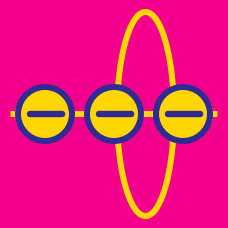Electricity and Magnetism

# Electric flux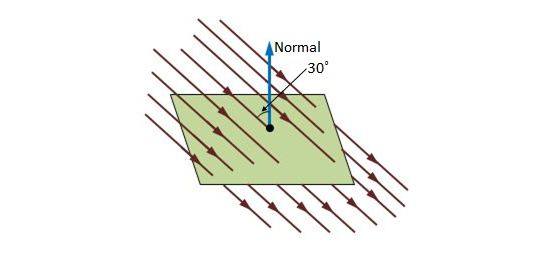A square surface with side length $3.7\text{ mm}$ is located in a uniform electric field with magnitude $E=2400\text{ N/C},$ as shown in the above figure. The angle between the normal to the surface and the electric field is $30^\circ.$ What is the electric flux through the surface, assuming that the normal is directed outward?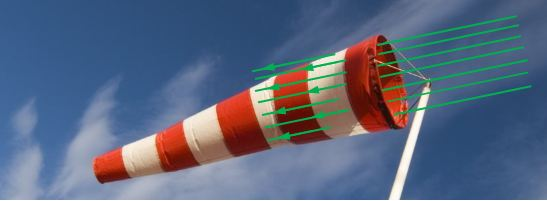An anemoscope is a device invented to show the direction of the wind, or to foretell a change of wind direction or weather. Consider a situation where an anemoscope is in a uniform electric field of magnitude $E=4.5\text{ mN/C},$ as depicted in the above figure. If the rim of the anemoscope is a circle with radius $r=13\text{ cm},$ and is perpendicular to the electric field, what is the magnitude of the electric flux through the fabric of the anemoscope?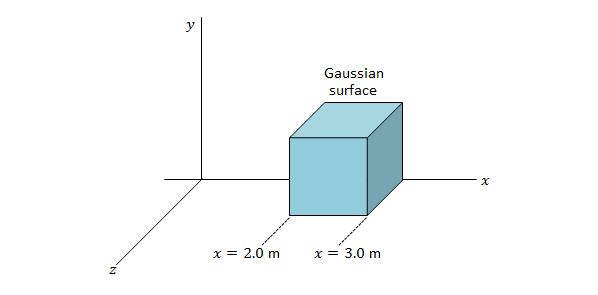A Gaussian cube of edge length $1.0\text{ m}$ is located in the $xyz$-space, as shown in the above figure. If the electric field in this space is expressed as $\overrightarrow{E}=4.0 \hat{i}-3.0(y^2+3.0) \hat{j} \text{ N/C},$ what is the electric flux through the top face of the Gaussian cube?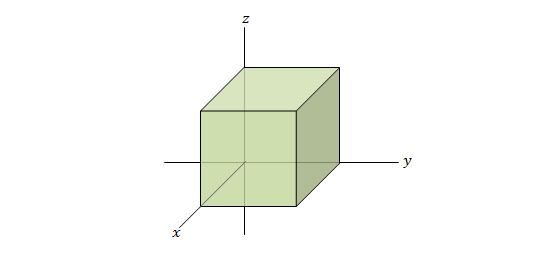A cube with edge length $0.90\text{ m}$ is located in the $xyz$-space as shown in the above figure. If the uniform electric field in the space is given by $-4.00 \hat{j} \text{ N/C},$ what is the electric flux through the right face of the cube?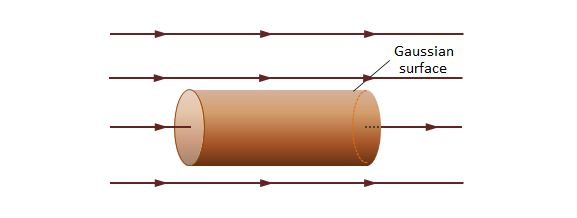The above figure shows a Gaussian surface, which is in the form of a cylinder of radius $R.$ The Gaussian surface is located in a uniform electric field $\overrightarrow{E},$ where the axis of the cylinder is parallel to the electric field. What is the flux $\Phi$ of the electric field through this Gaussian surface, assuming that $A$ in the answer denotes the cap's area $\pi R^2?$

×